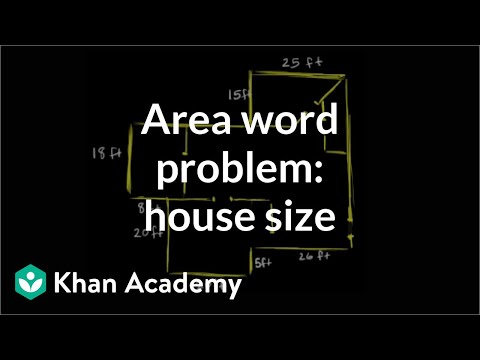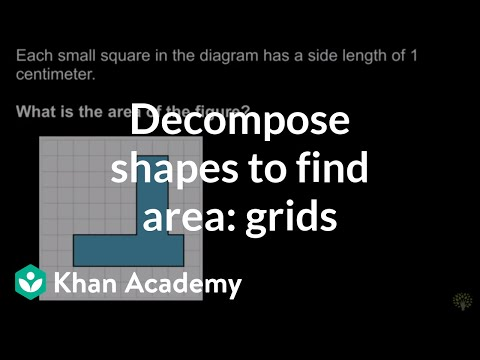Video

# Decomposing shapes to find area: add (Full video)

Description: Lindsay finds the area of an irregular shape by decomposing it into 3 rectangles and adding the area of the rectangles. So down here we have this one, two, three, four, five, six, seven, eight, nine, 10-sided figure, and we want to know its area, how many square meters does this figure cover? And we have some measurements, that seems helpful, but what's not too helpful to me is I don't know the special trick to find the area of a 10-sided figure so I've got to think about what I do know and what I do know is the way to find area of a rectangle. So what we did is, we broke this up or decomposed it into three rectangles and now if I find out how much space this purple one covers, and the blue one and the green one, if I combine those, that would tell me the area of the entire figure, how much space the entire figure covers.

### Other videos you might be interested in### Area word problem: house size (Full video)